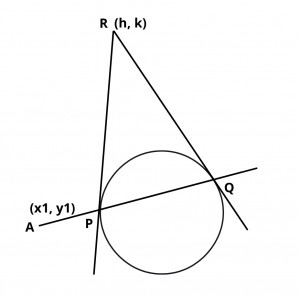# Pole and Polar of a Circle Equation

Here you will learn what is the pole and polar of a circle and pole of given line with respect to a circle.

Let’s begin –

## Pole and Polar of a Circle

Let any straight line through the given point A$$(x_1, y_1)$$ intersects the given circle S = 0 in two points P and Q and if the tangent of the circle at P and Q meet at the point R then the locus of point R is called polar of point A and point A is called the pole, with respect to the given circle.

## Equation of the Polar of Point

The equation of the polar of point $$(x_1, y_1)$$ with respect to circle $$x^2 + y^2$$ = $$a^2$$ is$$xx_1 + yy_1$$ = $$a^2$$

Proof : Let APQ is a chord given in figure which passes through the point A$$(x_1, y_1)$$ which intersects the circle at points P and Q and the tangents are drawn at points P and Q meet at point R (h, k) then the equation of PQ the chord of contact is $$x_1h + y_1k$$ = $$a^2$$

$$\therefore$$  Locus of point S(h, k) is $$xx_1 + yy_1$$ = $$a^2$$  which is the equation of the polar.

Note :

(i)  If point is outside the circle then the equation of polar and chord of contact is same. So the chord of contact is polar.

(ii)  If point is inside the circle then chord of contact does not exist but polar exist.

(iii)  If point lies on the circle then polar, chord of contact and tangent on that point are same.

(iv)  If polar of P w.r.t a circle passes through the point Q, then the polar of point Q will pass through P and hence P and Q are conjugate points of each other w.r.t the given circle.

(v)  If pole of a line w.r.t a circle lies on second line. Then pole of second line lies on first line and hence both lines are conjugate lines of each other w.r.t the given circle.

## Pole of a given line with respect to a circle

To find the pole of a line we assume the coordinates of the pole then from these coordinates we find the polar. This polar and the given line represent the same line.

Then by comparing the coefficients of similar terms we can get the coordinates of the pole.

The pole of line lx + my + n = 0 w.r.t circle $$x^2 + y^2$$ = $$a^2$$  will be

$$({-la^2\over n}, {-ma^2\over n})$$